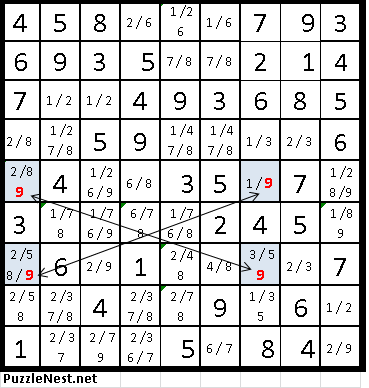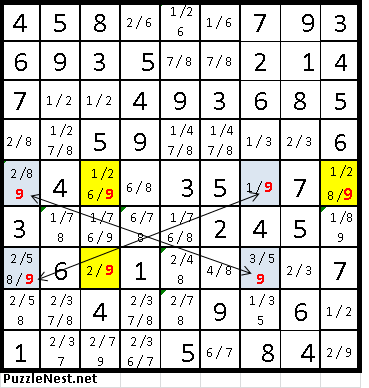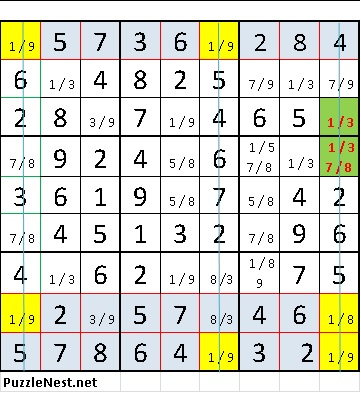# Advanced Sudoku tipsIf you solved few Sudoku puzzles and got to the point where whatever you tried, you simply could not finish the puzzle, then it is a sign you need some advance Sudoku techniques to improve your solving skills,

The techniques below will help you in reducing the number of candidates that can go into a cell or in some cases, assist you to eliminate some candidates and find the specific number that will go into that cell. These techniques are more elaborate than the simple tips we provide in the beginners tips (see here) and they require focus, concentration and the ability to recognize these strategy patterns.

Here are few advance pattern recognition strategies

1.   The X-Wing

This strategy helps us to eliminate some choices from penciled possibilities. This strategy consists of finding 2 rows and 2 columns where each pivot point contains a matching candidate number and forming a square or rectangular if connected.  Now if any of the rows or columns that form the square or rectangular contains the same number candidate anywhere else on the row or column that form the square or rectangular, then it can be eliminated. This technique is named X-wing because the candidate matching numbers are diagonally across each other within the square or rectangle.

Let’s look at an example:In this example, the penciled number 9 (marked in red) is positioned in row 5 in location R5C1 (Row 5, Column 1) and R5C7 and also in row 7 in positions R7C1 and R7C5. Therefore row 5 and 7 form a rectangle with column 1 and 7 with the optional candidate 9. These 4 cells are marked in blue.in the example above. You can notice they form a rectangular. This strategy is called X-Wing because the candidate matching numbers (in this case 9) are diagonally across each other within the rectangular and forming an X. Once we identify this form, and since the number 9 can appear only once in each row or column, then every other case of the candidate number 9 can be eliminated from other cells within the same row and column forming the rectangle. In the example below – we highlight in red all the candidate number 9 that now can be eliminated and highlight the box in yellow. As you can see, in box R7C3 once we eliminate the candidate number 9, we are left with the only option, which in this example is the number 2.It takes a bit of practice to identify this form, and it is fairly common in the tougher puzzles. Once you identify this form, it will help you to eliminate number candidates in few cells and at times even finalize a candidate number (like the number 2 after eliminating the candidate number 9 in cell R7C3.in the above example)

This technique requires the player to pay attention to this format and adopt a bird’s eye view yet finding the x-wing format can be instrumental in advancing your skillset and help in resolving more complex Sudoku puzzles.

2.   The Swordfish

If the X-wing technique consists of finding 2 rows and 2 columns where each pivot point contains a matching candidate number, the Swordfish technique is like the X-Wing technique on steroids and consists of finding 3 rows and 3 columns where each cross point between a row and column contains a matching candidate number.

Like the x-wing, this technique helps to eliminate some number candidates. Let’s look at an example to make this technique clearerIn the example shown above, a Sudoku Swordfish pattern happens in rows 1, 8, and 9.( highlight in light blue) The three columns are 1, 6, and 9. (A blue line cross it) Each row has the number 1 in only two cells in this case. In row 1 the cells are R1C1, and R1C6, In row 8, the cells are R8C1, and R8C9. In row 9, the cells are R9C6 and R9C9. These cells are highlighted in yellow. The number 1 can only reside in three of the six highlighted cells

We do know that it must reside in three of the six positions highlighted. (They are locked pairs.) Therefore it is safe to eliminate the 1s from all three columns (or rows) except for the six highlighted positions. In the example above, the two cells in red (highlight in Green) may have the number 1 removed from them safely. Once you removed the 1s, you now expose a naked single 3 in R3C9.

This is not an easy formation to find, and it takes practice and focus to recognize the pattern and find it, however, it is an effective method to eliminate some candidate numbers (in our example it is the number 1) and it is another effective strategy in your advance strategy toolbox to make the process of completion and solving the puzzle easier.